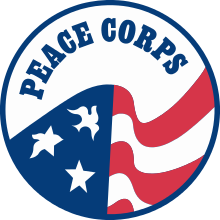The Peace Corps Bulgarian CoursesBulgarian

Introduction to the Bulgarian language

 Lesson 1 Lesson 2 Lesson 3 Lesson 4 Lesson 5 Lesson 6 Lesson 7 Lesson 8 Lesson 9 Lesson 10 Lesson 11 Lesson 12 Lesson 13 Lesson 14 Lesson 15 Lesson 16 Lesson 17